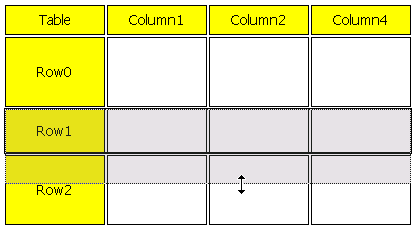# [Eclipse]GEF入门系列（十、表格的一个实现）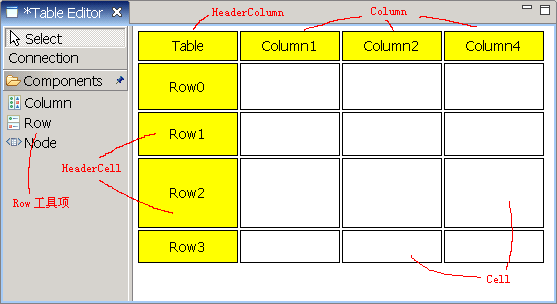tool = new CombinedTemplateCreationEntry("Row", "Create a new Row", HeaderCell.class, new SimpleFactory(HeaderCell.class), CbmPlugin.getImageDescriptor(IConstants.IMG_ROW), null);

protected void showLayoutTargetFeedback(Request request) {
super.showLayoutTargetFeedback(request);
// Expand feedback line's width
Diagram diagram = (Diagram) getHost().getParent().getModel();
Column column = (Column) getHost().getModel();
Point p2 = getLineFeedback().getPoints().getPoint(1);
p2.x = p2.x + (diagram.getColumns().size() - 1) * (column.getWidth() + IConstants.COLUMN_SPACING);
getLineFeedback().setPoint(p2, 1);
}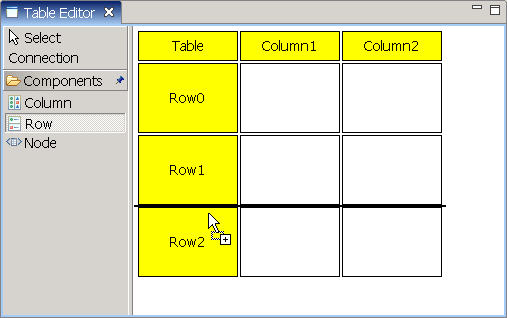public class RowMoveHandle extends MoveHandle {
public RowMoveHandle(GraphicalEditPart owner, Locator loc) {
super(owner, loc);
}
public RowMoveHandle(GraphicalEditPart owner) {
super(owner);
}
//计算得到选中行所占的位置，传给MoveHandleLocator作为参考
public Locator getLocator() {
IFigure refFigure = new Figure();
translateToAbsolute(rect);
refFigure.setBounds(rect);
return new MoveHandleLocator(refFigure);
}
}

public Rectangle getRowBound(){
Rectangle rect = getFigure().getBounds().getCopy();
Diagram diagram = (Diagram) getParent().getParent().getModel();
Column column = (Column) getParent().getModel();
rect.setSize(diagram.getColumns().size() * column.getWidth() + (diagram.getColumns().size() - 1) * IConstants.COLUMN_SPACING, rect.getSize().height);
return rect;
}

protected List createSelectionHandles() {
List l = new ArrayList();
//四周的黑色边框
//下方的控制点
return l;
}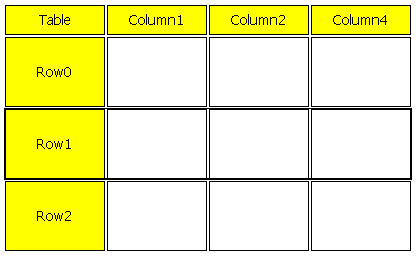public class RowResizeHandle extends ResizeHandle {
public RowResizeHandle(GraphicalEditPart owner, int direction) {
super(owner, direction);
//改变控制点的尺寸，使之变成一条线
}
public RowResizeHandle(GraphicalEditPart owner, Locator loc, Cursor c) {
super(owner, loc, c);
}
//缺省实现里控制点有描边，我们不需要，所以覆盖这个方法
public void paintFigure(Graphics g) {
Rectangle r = getBounds();
g.setBackgroundColor(getFillColor());
g.fillRectangle(r.x, r.y, r.width, r.height);
}
//与前面RowMoveHandle类似，但返回RelativeHandleLocator以使线显示在图形下方
public Locator getLocator() {
IFigure refFigure = new Figure();
translateToAbsolute(rect);
refFigure.setBounds(rect);
return new RelativeHandleLocator(refFigure, PositionConstants.SOUTH);
}
//不论是否为主选择，都使用黑色填充
protected Color getFillColor() {
return ColorConstants.black;
}
}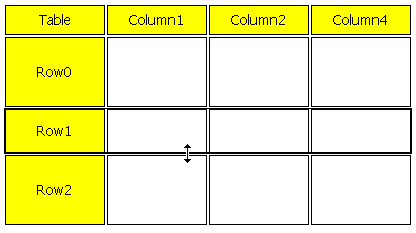protected Rectangle getInitialFeedbackBounds() {
}